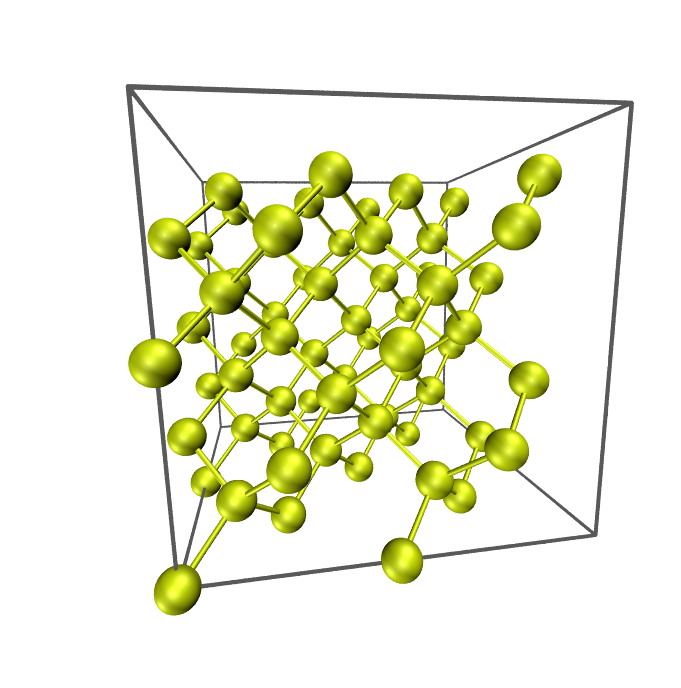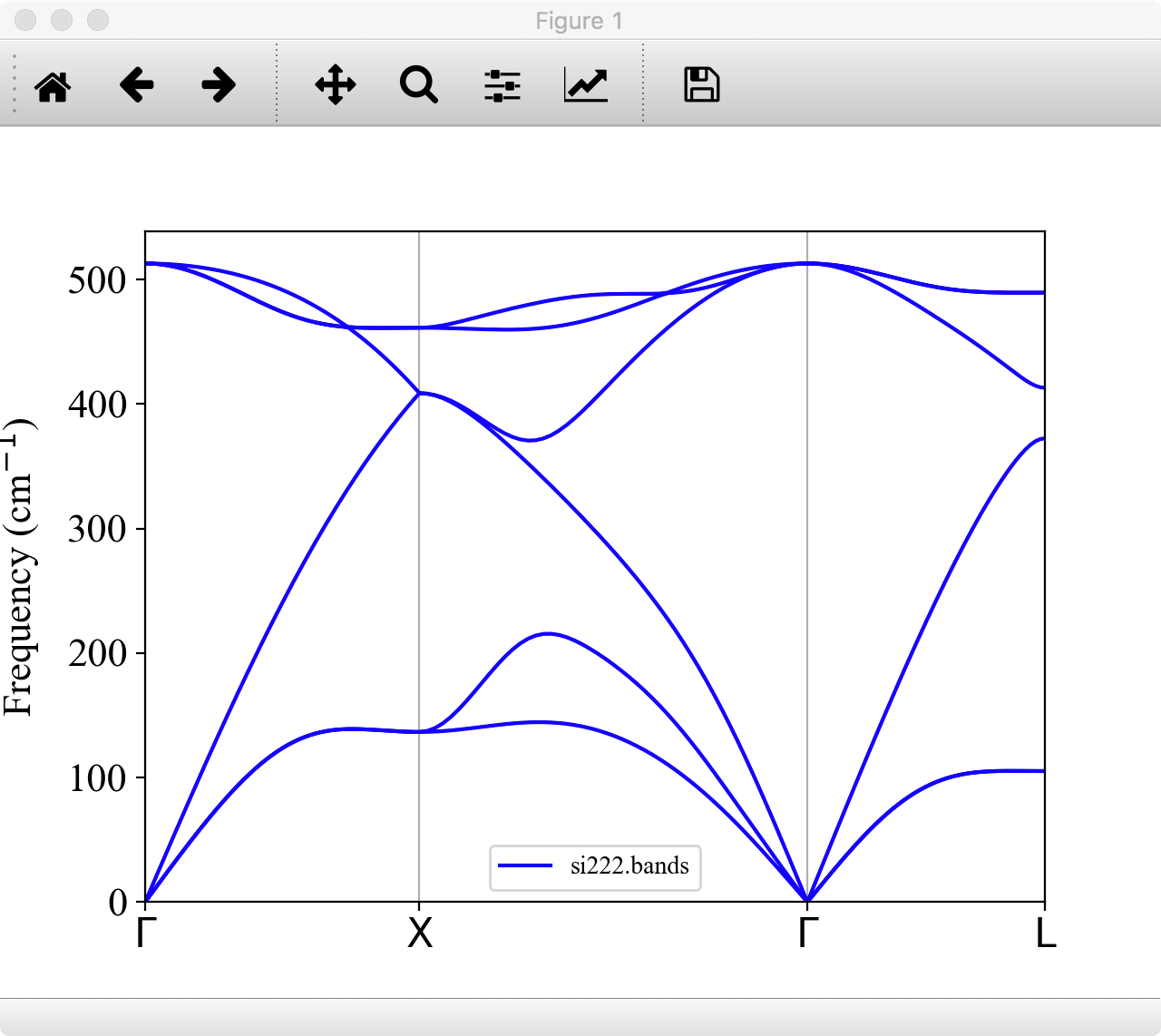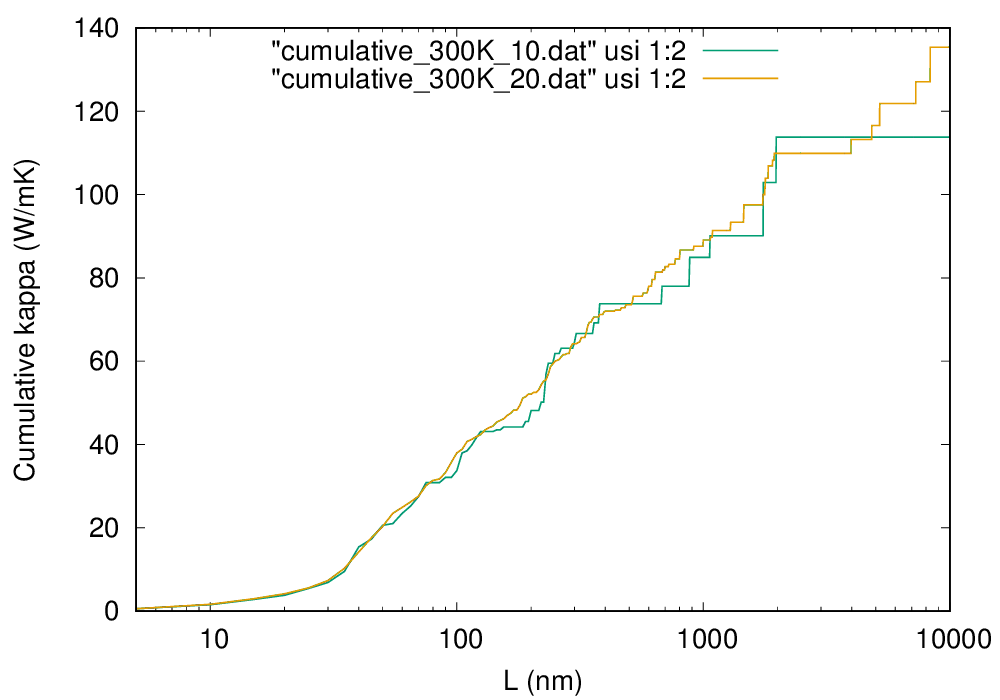# 7. Tutorial¶

Input files prepared for this tutorial are located in the example/ directory of the ALAMODE package.

## 7.1. Silicon¶Silicon. 2x2x2 conventional supercell

In the following, (anharmonic) phonon properties of bulk silicon (Si) are calculated by a 2x2x2 conventional cell containing 64 atoms.

### 1. Get displacement patterns by alm¶

Change directory to example/Si and open file si_alm.in. This file is an input for the code alm which estimate interatomic force constants (IFC) by least square fitting. In the file, the crystal structure of a 2x2x2 conventional supercell of Si is specified in the &cell and the &position fields as the following:

&general
PREFIX = si222
MODE = suggest
NAT = 64; NKD = 1
KD = Si
/

&interaction
NORDER = 1  # 1: harmonic, 2: cubic, ..
/

&cell
20.406 # factor in Bohr unit
1.0 0.0 0.0 # a1
0.0 1.0 0.0 # a2
0.0 0.0 1.0 # a3
/

&cutoff
Si-Si None
/

&position
1 0.0000000000000000 0.0000000000000000 0.0000000000000000
1 0.0000000000000000 0.0000000000000000 0.5000000000000000
1 0.0000000000000000 0.2500000000000000 0.2500000000000000
1 0.0000000000000000 0.2500000000000000 0.7500000000000000
1 0.0000000000000000 0.5000000000000000 0.0000000000000000
1 0.0000000000000000 0.5000000000000000 0.5000000000000000
1 0.0000000000000000 0.7500000000000000 0.2500000000000000


Replace the lattice constant of the supercell (20.406 Bohr) by your own value.

Then, execute alm

$alm si_alm.in > si_alm.log1  which creates a file si222.pattern_HARMONIC in the working directory. In the pattern file, suggested displacement patterns are defined in Cartesian coordinates. As you can see in the file, there is only one displacement pattern for harmonic IFCs of bulk Si. ### 2. Calculate atomic forces for the displaced configurations¶ Next, calculate atomic forces for all the displaced configurations defined in si222.pattern_HARMONIC. To do so, you first need to decide the magnitude of displacements $$\Delta u$$, which should be small so that anharmonic contributions are negligible. In most cases, $$\Delta u \sim 0.01$$ Å is a reasonable choice. Then, prepare input files necessary to run an external DFT code for each configuration. Since this procedure is a little tiresome, we provide a subsidiary Python script for VASP, Quantum-ESPRESSO (QE), and xTAPP. Using the script displace.py in the tools/ directory, you can generate the necessary input files as follows: QE $ python displace.py --QE=si222.pw.in --mag=0.01 -pf si222.pattern_HARMONIC


VASP

$python displace.py --VASP=POSCAR.orig --mag=0.01 -pf si222.pattern_HARMONIC  xTAPP $ python displace.py --xTAPP=si222.cg --mag=0.01 -pf si222.pattern_HARMONIC


OpenMX

$python displace.py --OpenMX=si222.dat --mag=0.01 -pf si222.pattern_HARMONIC  The --mag option specifies the displacement length in units of Angstrom. You need to specify an input file with equilibrium atomic positions either by the --QE, --VASP, --xTAPP, --OpenMX or --LAMMPS. Then, calculate atomic forces for all the configurations. This can be done with a simple shell script as follows: #!/bin/bash # Assume we have 20 displaced configurations for QE [disp01.pw.in,..., disp20.pw.in]. for ((i=1;i<=20;i++)) do num=echo$i | awk '{printf("%02d",$1)}' mkdir${num}
cd ${num} cp ../disp${num}.pw.in .
pw.x < disp${num}.pw.in > disp${num}.pw.out
cd ../
done


Important

In QE, you need to set tprnfor=.true. to print out atomic forces.

The next step is to collect the displacement data and force data by the Python script extract.py (also in the tools/ directory). This script can extract atomic displacements, atomic forces, and total energies from multiple output files as follows:

QE

$python extract.py --QE=si222.pw.in *.pw.out > DFSET_harmonic  VASP $ python extract.py --VASP=POSCAR.orig vasprun*.xml > DFSET_harmonic


xTAPP

$python extract.py --xTAPP=si222.cg *.str > DFSET_harmonic  OpenMX $ python extract.py --OpenMX=si222.dat *.out > DFSET_harmonic


In the above examples, atomic displacements and corresponding atomic forces of all the configurations are merged as DFSET_harmonic. These files will be used in the following fitting procedure as DFSET (See Format of DFSET).

Note

For your convenience, we provide the DFSET_harmonic file in the reference/ subdirectory. These files are generated by the Quantum-ESPRESSO package with --mag=0.02. You can proceed to the next step by copying these files to the working directory.

### 3. Estimate force constants by fitting¶

Edit the file si_alm.in to perform least-square fitting. Change the MODE = suggest to MODE = optimize as follows:

&general
PREFIX = si222
MODE = optimize   # <-- here
NAT = 64; NKD = 1
KD = Si
/


Also, add the &optimize field as:

&optimize
DFSET = DFSET_harmonic
/


Then, execute alm again

$alm si_alm.in > si_alm.log2  This time alm extract harmonic IFCs from the given displacement-force data set (DFSET_harmonic). You can find files si222.fcs and si222.xml in the working directory. The file si222.fcs contains all IFCs in Rydberg atomic units. You can find symmetrically irreducible sets of IFCs in the first part as:  *********************** Force Constants (FCs) *********************** * Force constants are printed in Rydberg atomic units. * * FC2: Ry/a0^2 FC3: Ry/a0^3 FC4: Ry/a0^4 etc. * * FC?: Ry/a0^? a0 = Bohr radius * * * * The value shown in the last column is the distance * * between the most distant atomic pairs. * ********************************************************************* ---------------------------------------------------------------------- Index FCs P Pairs Distance [Bohr] (Global, Local) (Multiplicity) ---------------------------------------------------------------------- *FC2 1 1 2.7613553e-01 1 1x 1x 0.000 2 2 -9.5252308e-04 2 1x 2x 10.203 3 3 -3.7574247e-04 2 1x 33x 10.203 4 4 8.8561868e-03 1 1x 3x 7.215 5 5 1.9633030e-03 1 1x 3y 7.215 6 6 -3.9798276e-03 1 1x 17x 7.215 7 7 -3.9080855e-03 1 1x 17z 7.215 8 8 8.2188178e-03 4 1x 6x 14.429 9 9 4.3878651e-05 4 1x 34x 14.429 10 10 -6.7358757e-02 1 1x 9x 4.418 11 11 -4.7360832e-02 1 1x 9y 4.418 12 12 1.0670028e-03 1 1x 10x 8.460 13 13 -8.2098635e-04 1 1x 10y 8.460 14 14 -6.9742830e-04 1 1x 10z 8.460 15 15 2.1135669e-04 1 1x 26x 8.460 16 16 -4.3031422e-03 1 1x 11x 11.118 17 17 5.0759340e-04 1 1x 11y 11.118 18 18 -4.7178438e-04 1 1x 25x 11.118 19 19 -4.7518256e-04 1 1x 25z 11.118 20 20 -7.4138963e-04 2 1x 20x 12.496 21 21 1.2900836e-03 2 1x 20y 12.496 22 22 -1.7263732e-04 2 1x 20z 12.496 23 23 6.4515756e-05 2 1x 35x 12.496 24 24 3.5369980e-04 1 1x 28x 13.254 25 25 -5.1486930e-04 1 1x 28y 13.254  Harmonic IFCs $$\Phi_{\mu\nu}(i,j)$$ in the supercell are given in the third column and the multiplicity $$P$$ is the number of times each interaction $$(i, j)$$ occurs within the given cutoff radius. For example, $$P = 2$$ for the pair $$(1x, 2x)$$ because the distance $$r_{1,2}$$ is exactly the same as the distance $$r_{1,2'}$$ where the atom 2’ is a neighboring image of atom 2 under the periodic boundary condition. If you compare the magnitude of IFCs, the values in the third column should be divided by $$P$$. In the log file si_alm.log2, the fitting error is printed. Try $ grep "Fitting error" si_alm.log2
Fitting error (%) : 0.567187


The other file si222.xml contains crystal structure, symmetry, IFCs, and all other information necessary for subsequent phonon calculations.

### 4. Calculate phonon dispersion and phonon DOS¶

Open the file si_phband.in and edit it for your system.

&general
PREFIX = si222
MODE   = phonons
FCSXML = si222.xml

NKD = 1; KD = Si
MASS = 28.0855
/

&cell
10.203
0.0 0.5 0.5
0.5 0.0 0.5
0.5 0.5 0.0
/

&kpoint
1  # KPMODE = 1: line mode
G 0.0 0.0 0.0 X 0.5 0.5 0.0 51
X 0.5 0.5 1.0 G 0.0 0.0 0.0 51
G 0.0 0.0 0.0 L 0.5 0.5 0.5 51
/



Please specify the XML file you obtained in step 3 by the FCSXML-tag as above. In the &cell-field, you need to define the lattice vector of a primitive cell. In phonon dispersion calculations, the first entry in the &kpoint-field should be 1 (KPMODE = 1).

Then, execute anphon

$anphon si_phband.in > si_phband.log  which creates a file si222.bands in the working directory. In this file, phonon frequencies along the given reciprocal path are printed in units of cm-1 as: # G X G L # 0.000000 0.615817 1.486715 2.020028 # k-axis, Eigenvalues [cm^-1] 0.000000 1.097373e-10 1.097373e-10 1.097373e-10 5.129224e+02 5.129224e+02 5.129224e+02 0.012316 6.009520e+00 6.009520e+00 1.022526e+01 5.128286e+02 5.128286e+02 5.128923e+02 0.024633 1.200989e+01 1.200989e+01 2.044420e+01 5.125479e+02 5.125479e+02 5.128019e+02 0.036949 1.799191e+01 1.799191e+01 3.065053e+01 5.120824e+02 5.120824e+02 5.126510e+02 0.049265 2.394626e+01 2.394626e+01 4.083799e+01 5.114353e+02 5.114353e+02 5.124391e+02 0.061582 2.986346e+01 2.986346e+01 5.100034e+01 5.106114e+02 5.106114e+02 5.121656e+02 0.073898 3.573381e+01 3.573381e+01 6.113142e+01 5.096167e+02 5.096167e+02 5.118299e+02  You can plot the phonon dispersion relation with gnuplot or any other plot software. For visualizing phonon dispersion relations, we provide a Python script plotband.py in the tools/ directory (Matplotlib is required.). Try $ python plotband.py si222.bands


Then, the phonon dispersion is displayed as follows:You can save the figure as png, eps, or other formats from this window. You can also change the energy unit of phonon frequency from cm-1 to THz or meV by the --unit option. For more detail of the usage of plotband.py, type

$python plotband.py -h  Next, let us calculate the phonon DOS. Copy si_phband.in to si_phdos.in and edit the &kpoint field as follows: &kpoint 2 # KPMODE = 2: uniform mesh mode 20 20 20 /  Then, execute anphon $ anphon si_phdos.in > si_phdos.log


This time, anphon creates files si222.dos and si222.thermo in the working directory, which contain phonon DOS and thermodynamic functions, respectively. For visualizing phonon DOS and projected DOSs, we provide a Python script plotdos.py in the tools/ directory (Matplotlib is required.). The command

$python plotdos.py --emax 550 --nokey si222.dos  will show the phonon DOS of Si by a pop-up window:To improve the resolution of DOS, try again with a denser $$k$$ grid and a smaller DELTA_E value. ### 5. Estimate cubic IFCs for thermal conductivity¶ Copy file si_alm.in to si_alm2.in. Edit the &general, &interaction, and &cutoff fields of si_alm2.in as the following: &general PREFIX = si222_cubic MODE = suggest NAT = 64; NKD = 1 KD = Si /  Change the PREFIX from si222 to si222_cubic and set MODE to suggest. &interaction NORDER = 2 /  Change the NORDER tag from NORDER = 1 to NORDER = 2 to include cubic IFCs. Here, we consider cubic interaction pairs up to second nearest neighbors by specifying the cutoff radii as: &cutoff Si-Si None 7.3 /  7.3 Bohr is slightly larger than the distance of second nearest neighbors (7.21461 Bohr). Change the cutoff value appropriately for your own case. (Atomic distance can be found in the file si_alm.log.) Then, execute alm $ alm si_alm2.in > si_alm2.log


which creates files si222_cubic.pattern_HARMONIC and si222_cubic.pattern_ANHARM3.

Then, calculate atomic forces of displaced configurations given in the file si222_cubic.pattern_ANHARM3, and collect the displacement and force datasets to a file DFSET_cubic as you did for harmonic IFCs in Steps 3 and 4.

Note

Since making DFSET_cubic requires moderate computational resources, you can skip this procedure by copying file reference/DFSET_cubic to the working directory. The file we provide is generated by the Quantum-ESPRESSO package with --mag=0.04.

In si_alm2.in, change MODE = suggest to MODE = optimize and add the following:

&optimize
DFSET = DFSET_cubic
FC2XML = si222.xml # Fix harmonic IFCs
/


By the FC2XML tag, harmonic IFCs are fixed to the values in si222.xml. Then, execute alm again

$alm si_alm2.in > si_alm2.log2  which creates files si222_cubic.fcs and si222_cubic.xml. This time cubic IFCs are also included in these files. Note In the above example, we obtained cubic IFCs by least square fitting with harmonic IFCs being fixed to the value of the previous harmonic calculation. You can also estimate both harmonic and cubic IFCs simultaneously instead. To do this, merge DFSET_harmonic and DFSET_cubic as $ cat DFSET_harmonic DFSET_cubic > DFSET_merged


and change the &optimize field as the following:

&optimize
DFSET = DFSET_merged
/


### 6. Calculate thermal conductivity¶

Copy file si_phdos.in to si_RTA.in and edit the MODE and FCSXML tags as follows:

&general
PREFIX = si222
MODE = RTA
FCSXML = si222_cubic.xml

NKD = 1; KD = Si
MASS = 28.0855
/


In addition, change the $$k$$ grid density as:

&kpoint
2
10 10 10
/


Then, execute anphon as a background job

$anphon si_RTA.in > si_RTA.log &  Please be patient. This can take a while. When the job finishes, you can find a file si222.kl in which the lattice thermal conductivity is saved. You can plot this file using gnuplot (or any other plotting softwares) as follows: $ gnuplot
gnuplot> set logscale xy
gnuplot> set xlabel "Temperature (K)"
gnuplot> set ylabel "Lattice thermal conductivity (W/mK)"
gnuplot> plot "si222.kl" usi 1:2 w lpCalculated lattice thermal conductivity of Si (click to enlarge)

As you can see, the thermal conductivity diverges in $$T\rightarrow 0$$ limit. This occurs because we only considered intrinsic phonon-phonon scatterings in the present calculation and neglected phonon-boundary scatterings which are dominant in the low-temperature range. The effect of the boundary scattering can be included using the python script analyze_phonons.py in the tools directory:

$gnuplot gnuplot> set logscale x gnuplot> set xlabel "L (nm)" gnuplot> set ylabel "Cumulative kappa (W/mK)" gnuplot> plot "cumulative_300K_10.dat" using 1:2 w lpCumulative thermal conductivity of Si at 300 K (click to enlarge) To draw a smooth line, you have to use a denser $$q$$ grid as shown in the figure by the orange line, which are obtained with $$20\times 20\times 20\ q$$ points. #### Thermal conductivity spectrum¶ To calculate the spectrum of thermal conductivity, modify the si_RTA.in as follows: &general PREFIX = si222 MODE = RTA FCSXML = si222_cubic.xml NKD = 1; KD = Si MASS = 28.0855 EMIN = 0; EMAX = 550; DELTA_E = 1.0 # <-- frequency range / &cell 10.203 0.0 0.5 0.5 0.5 0.0 0.5 0.5 0.5 0.0 / &kpoint 2 10 10 10 / &analysis KAPPA_SPEC = 1 # compute spectrum of kappa /  The frequency range is specified with the EMIN, EMAX, and DELTA_E tags, and the KAPPA_SPEC = 1 is set in the &analysis field. Then, execute anphon again $ anphon si_RTA.in > si_RTA2.log


After the calculation finishes, you can find the file si222.kl_spec which contains the spectra of thermal conductivity at various temperatures. You can plot the data at room temperature as follows:

$awk '{if ($1 == 300.0) print $0}' si222.kl_spec > si222_300K_10.kl_spec$ gnuplot
gnuplot> set xlabel "Frequency (cm^{-1})"
gnuplot> set ylabel "Spectrum of kappa (W/mK/cm^{-1})"
gnuplot> plot "si222_300K_10.kl_spec" using 2:3 w l lt 2 lw 2The spectrum of thermal conductivity of Si at 300 K (click to enlarge)

In the above figure, the computational result with $$20\times 20\times 20\ q$$ points is also shown by the dashed line. From the figure, we can observe that low-energy phonons below 200 cm$$^{-1}$$ account for more than 80% of the total thermal conductivity at 300 K.

## 7.2. Silicon with LAMMPS¶

Here, we demonstrate how to use ALAMODE together with LAMMPS. All input files can be found in the example/Si_LAMMPS directory. Before starting the tutorial, please build the LAMMPS code (e.g. lmp_serial).

As a simple example, we calculate phonon dispersion curves of Si using the Stillinger-Weber (SW) potential implemented in LAMMPS. First, you need to make two input files for LAMMPS: in.sw and Si222.lammps (file name is arbitrary, though). in.sw is the main input file for LAMMPS, in which the type of the empirical force field is defined as follows:

units           metal
atom_style      atomic
boundary        p p p

pair_style      sw
pair_coeff 	* * Si.sw Si

dump            1 all custom 1 XFSET id xu yu zu fx fy fz
dump_modify     1 format float "%20.15f"
run             0



In the file Si222.lammps, the lattice vectors and atomic positions of a relaxed supercell structure are defined as follows:

# Structure data of Si (2x2x2 conventional)

64 atoms
1 atom types

0.000000   10.800000   xlo xhi
0.000000   10.800000   ylo yhi
0.000000   10.800000   zlo zhi
0.000000    0.000000    0.000000   xy xz yz

Masses

1 28.085

Atoms

1  1      0.000000 0.000000 0.000000
2  1      0.000000 0.000000 5.400000
3  1      0.000000 2.700000 2.700000
4  1      0.000000 2.700000 8.100000
5  1      0.000000 5.400000 0.000000
6  1      0.000000 5.400000 5.400000
7  1      0.000000 8.100000 2.700000
8  1      0.000000 8.100000 8.100000
9  1      1.350000 1.350000 1.350000
10  1      1.350000 1.350000 6.750000
11  1      1.350000 4.050000 4.050000
12  1      1.350000 4.050000 9.450000
13  1      1.350000 6.750000 1.350000
14  1      1.350000 6.750000 6.750000


Next, please generate a set of structure files for displaced configurations using the python script:

$python displace.py --LAMMPS=Si222.lammps --mag=0.01 --prefix harm -pf si222.pattern_HARMONIC$ python displace.py --LAMMPS=Si222.lammps --mag=0.04 --prefix cubic -pf si222.pattern_ANHARM3


The pattern files can be generated by the alm code as decribed here. The above commands create harm1.lammps and cubic[01-20].lammps structure files. Then, run the following script and calculate atomic forces for the generated structures. This should finish in a few seconds.

#!/bin/bash

cp harm1.lammps tmp.lammps
lmp_serial < in.sw > log.lammps
cp XFSET XFSET.harm1

for ((i=1;i<=20;i++))
do
num=echo $i | awk '{printf("%02d",$1)}'
cp cubic${num}.lammps tmp.lammps lmp_serial < in.sw > log.lammps cp XFSET XFSET.cubic${num}
done


After the force calculations are finished, displacement and force data sets can be generated as follows:

$python extract.py --LAMMPS=Si222.lammps XFSET.harm1 > DFSET_harmonic$ python extract.py --LAMMPS=Si222.lammps XFSET.cubic* > DFSET_cubic


Then, using these files and following exactly the same procedure as the last tutorial section, you can calculate phonons and thermal conductivity of Si using the SW potential.